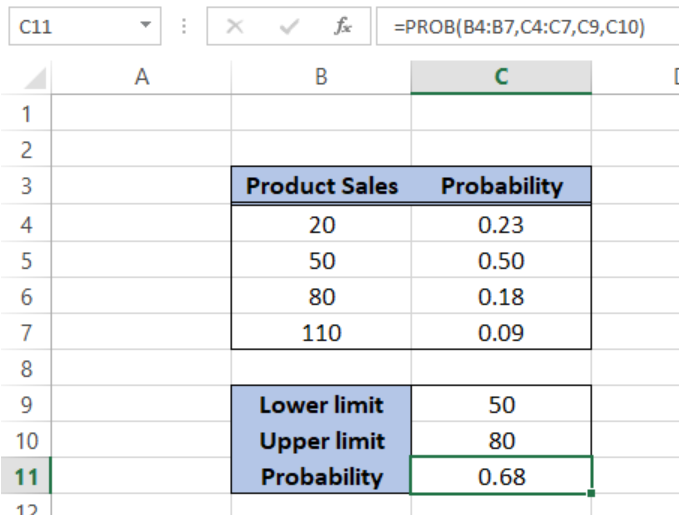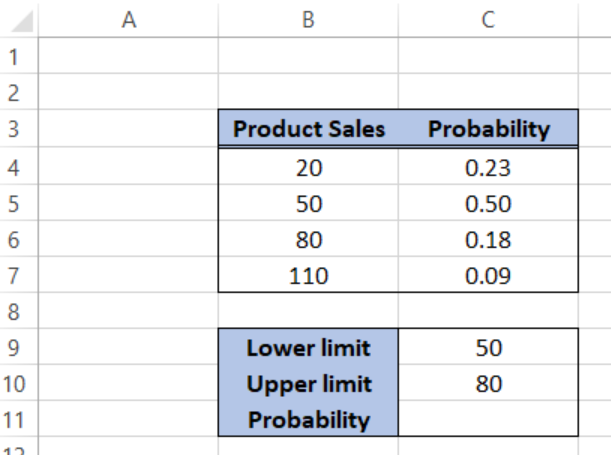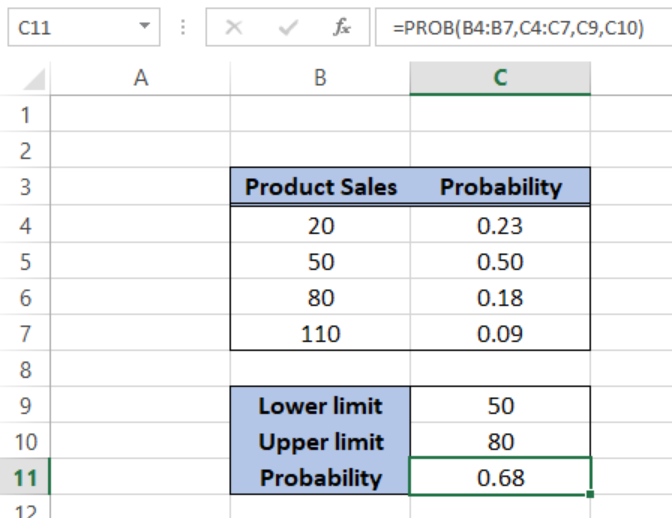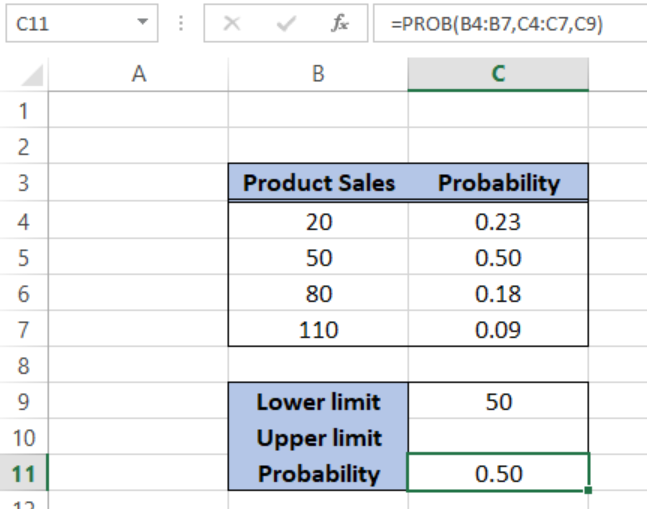Get instant live expert help with Excel or Google Sheets“My Excelchat expert helped me in less than 20 minutes, saving me what would have been 5 hours of work!”

#### Post your problem and you’ll get Expert help in seconds.

Your message must be at least 40 characters
Our professional Expert are available now. Your privacy is guaranteed.

# How to calculate probability in Excel – Excelchat

Probability is defined as the likelihood for which an event is probable, or likely to happen.  It is measured through the ratio of favorable events to the total number of possible cases. Excel has a built-in formula to calculate probability through the PROB function.Figure 1.  Final result:  Calculate Probability

## Syntax of PROB

`=PROB(range, prob_range, [lower_limit], [upper_limit])`

• range – the range of numeric values containing our data
• prob_range – the range of probabilities for each corresponding value in our range
• lower_limit – optional; the lower limit of the values for which we want to calculate the probability
• upper_limit – optional; the upper limit of the values for which we want to calculate the probability

## How to calculate probability?

In order to obtain reliable probability results, we should be able to prepare the data prior to calculation.

### Prepare our data

Below table contains a list of Product Sales and their corresponding probabilities.  The sum of all probabilities should be equal to 100. Otherwise, the PROB function returns the #NUM! error.Figure 2.  Sample data to calculate probability

We want to calculate the probability that a product sales is between 50 and 80.

### Calculating probability

To perform the calculation, we enter this formula in cell C11

`=PROB(B4:B7,C4:C7,C9,C10)`

Where B4:B7 is the range containing the values for product sales, C4:C7 contains the probabilities for each sales quantity, C9 is the lower limit of 50 while C10 is the upper limit of 80.Figure 3.  Entering the probability formula

As a result, the probability in cell C11 is 0.68 or 68%, which is the probability that product sales is between 50 and 80.

## Calculate the probability without upper limit

If there is no upper limit, the PROB function returns the probability of being equal to the lower limit only.

### Example:

If we omitted the upper limit in our formula, the result in cell C11 is 0.50 or 50%, which is also the probability of product sales being equal to 50.Figure 4.  Probability formula without upper limit

## Instant Connection to an Excel Expert

Most of the time, the problem you will need to solve will be more complex than a simple application of a formula or function. If you want to save hours of research and frustration, try our live Excelchat service! Our Excel Experts are available 24/7 to answer any Excel question you may have. We guarantee a connection within 30 seconds and a customized solution within 20 minutes.